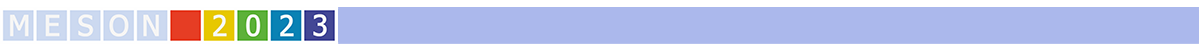#MESON2023

Jun 22 – 27, 2023
Auditorium Maximum
Europe/Warsaw timezone

## A study of $K^{-}d$ and $K^{+}d$ interactions via femtoscopy technique

Jun 24, 2023, 3:40 PM
20m
Conference room (Auditorium Maximum)

Parallel

### Speaker

Wioleta Rzęsa (Warsaw University of Technology)

### Description

The strong interaction between charged kaon and deuteron has previously been studied by scattering cross section measurements. However, due to the complexity of the system, some questions remain open at both experimental and theoretical levels. For example, the scattering lengths of the strong interaction of $K^{-}d$ and $K^{+}d$ have never been measured. Moreover, currently available theoretical calculations of this scattering parameter for $K^{-}d$ are largely based on input from kaonic hydrogen measurements. In the case of the scattering length of $K^{+}d$, there are no theoretical predictions published so far.

In this talk, the first measurements of the scattering lengths of $K^{+}d$ and $K^{-}d$ particle pairs are presented. The values of the scattering parameters are obtained using a femtoscopy technique, which is excellent for studying interactions between two particles with low relative momenta.

Collaboration ALICE

### Primary author

Wioleta Rzęsa (Warsaw University of Technology)